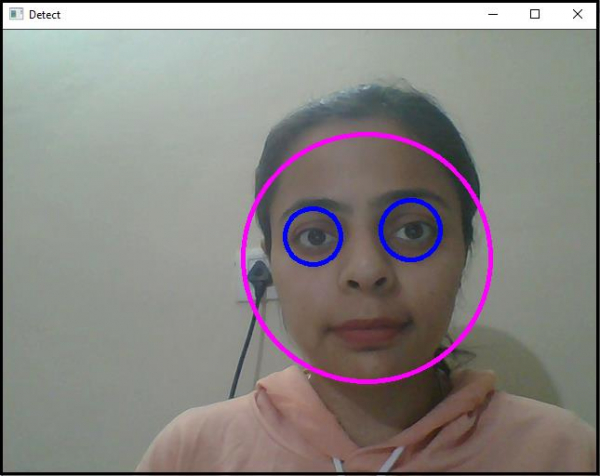# How to detect the eye in OpenCV using C++?

Here, we will learn how to detect the eye in OpenCV. We will use haarcascade_eye.xml classifier located in 'C:/opencv/sources/data/haarcascades' to detect the eyes. To detect the eyes, we need to add these headers.

The first header is <iostream>, and it is the header of C++ programming language. Reading writing images and user interface functionalities are defined in 'highgui' header. We need to add 'imgproc' header to enhance image quality, and we also use 'objdetect' header to detect face and eyes.

The following program to demonstrate how to detect and track eye in OpenCV.

## Example

#include<iostream>
#include<opencv2/highgui/highgui.hpp>
#include<opencv2/imgproc/imgproc.hpp>
#include<opencv2/objdetect/objdetect.hpp>
using namespace cv;
using namespace std;
int main() {
Mat frame;//declaring a matrix to video frame in it//
namedWindow("Detect");//Declaring a window to show our work//
VideoCapture image(0);//capturing video from default camera//
cout << "Couldn't load video from the source.Make sure your camera is working properly." << endl;
system("pause");
return 0;
}
double height = image.set(CAP_PROP_FRAME_HEIGHT, 480);//setting up height of each frame//
double width = image.set(CAP_PROP_FRAME_WIDTH, 640);//setting up width of each frame//
while (true) {
vector<Rect>faces;//Declaring a vector named faces//
for (int i = 0; i < faces.size(); i++){ //for locating the face
Point center(faces[i].x + faces[i].width * 0.5, faces[i].y + faces[i].height * 0.5);//getting the center of the face//
ellipse(frame, center, Size(faces[i].width * 0.5, faces[i].height * 0.5), 0, 0, 360, Scalar(255, 0, 255), 4, 8, 0);//draw an ellipse on the face//
Mat faceROI = frame(faces[i]);//Taking area of the face as Region of Interest for eyes//
vectoreyes;//declaring a vector named eyes//
eyes_cascade.detectMultiScale(faceROI, eyes, 1.1, 2, 0 | CASCADE_SCALE_IMAGE, Size(5, 5));//detect eyes in every face//
for (size_t j = 0; j < eyes.size(); j++){ //for locating eyes//
Point center(faces[i].x + eyes[j].x + eyes[j].width * 0.5, faces[i].y + eyes[j].y + eyes[j].height * 0.5);//getting the centers of both eyes//
int radius = cvRound((eyes[j].width + eyes[j].height) * 0.25);//declaring radius of the eye enclosing circles//
circle(frame, center, radius, Scalar(255, 0, 0), 4, 8, 0);//drawing circle around both eyes//
}
}
imshow("Detect", frame);//showing result in window named 'Detect'//
if (waitKey(30) == 27){ //wait time for each frame is 30 milliseconds//
break;
}
}
return 0;
}

## Output